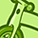# New to Qlik Sense

If you’re new to Qlik Sense, start with this Discussion Board and get up-to-speed quickly.

Announcements
Our May 2021 end-to-end product release from Data Integration to Data Analytics is out! READ DETAILS
cancel
Showing results for
Search instead for
Did you mean:Contributor II

## Qliksense countif for a result of a calculation

Hej,

I'm trying to calculate how many units is within our frames for turnover %. What I would like to accomplish is that data is aggregated on country level as % of positive answer to total number of units. On a unit level you should be able to see unit result. The span is 5-25%

Country: number of units in span/total number of units within a country

Unit: If in span display 'In span', else 'Not in span'

I tried this but it's not correct result. In my test data I have a unit1 in span (22%) and unit2 not in span (30%). The formula should show for a country 50% and if an user select unit1 then 'in' and for unit2 'not in' if both - nothing.

if( Count({<[BAL_TURNOVER_GRP FY19]={'YES'}>} CW_PERSNO_TM )/((Count({<[BAL_TURNOVER_GRP FY19]={'YES'}>} CW_PERSNO_Start)+Count({<[BAL_TURNOVER_GRP FY19]={'YES'}>} CW_PERSNO_End))/2)>=0.05

and

Count({<[BAL_TURNOVER_GRP FY19]={'YES'}>} CW_PERSNO_TM )/((Count({<[BAL_TURNOVER_GRP FY19]={'YES'}>} CW_PERSNO_Start)+Count({<[BAL_TURNOVER_GRP FY19]={'YES'}>} CW_PERSNO_End))/2)<=0.25,

Count(distinct BU),'')

or the below that works for single unit but not for a country level:

If((Count({<[BAL_TURNOVER_GRP FY19]={'YES'}>} CW_PERSNO_TM)
/
((Count({<[BAL_TURNOVER_GRP FY19]={'YES'}>} CW_PERSNO_Start)+
Count({<[BAL_TURNOVER_GRP FY19]={'YES'}>} CW_PERSNO_End))/2)>=0.05)

and
(Count({<[BAL_TURNOVER_GRP FY19]={'YES'}>} CW_PERSNO_TM)
/
((Count({<[BAL_TURNOVER_GRP FY19]={'YES'}>} CW_PERSNO_Start)+
Count({<[BAL_TURNOVER_GRP FY19]={'YES'}>} CW_PERSNO_End))/2)<=0.25),
'In span','Not in span')

Any ideas much appreciated?

Labels (2)

• ### Qlik Sense

0 Replies